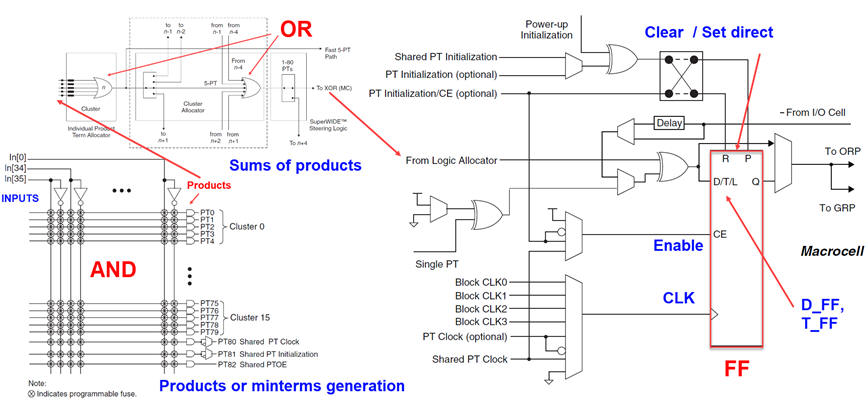﻿ Digital Circuits and Systems - Circuits i Sistemes Digitals (CSD) - EETAC - UPCBachelor's Degree in Telecommunications Systems and in Network EngineeringLecture 3 L5.3: Flip-flop specifications and applications [P5] - D-type, JK, and T flip-flops [21/4]

2.3.6.2. Data flip-flop (D_FF)

2.3.6.2.1. Function table, state diagram, timing diagram

This is the full tutorial on designing our D_FF. In the development section are presented two VHDL files that translate the behaviour of this component.

2.3.6.2.2. Deducing D_FF from RS_FF

As an alternative, we can add  a bit of theory using plan C2 to imagine D_FF from the previous RS_FF2.3.6.2.3. Commercial chip

A standard commercial D_FF is the 74HCT273.

2.3.6.3. JK flip-flop (JK_FF)

2.3.6.3.1. Function table, state diagram, timing diagram

This is the full tutorial on designing our JK_FF. In the development section are presented two VHDL files that translate the behaviour of this component.

2.3.6.3.2. Commercial chip

Classic chips of this kind are HEF4027B and 74HCT73.

2.3.6.4. Toggle flip-flop (T_FF)

2.3.6.4.1. Function table, state diagram, timing diagram

This is the full tutorial on designing our T_FF.

2.3.6.4.2. Frequency divider by two

This is simple and typical application if such component, imagining it a a CLK's frequency divider by 2. We will take advantage of this circuit when inventing  circuits in P8.

Flip-flops are basic building blocks for digital systems. They are very well documented.

Programmable logic devices (PLD) contain a flip-flop in its logic cell. For instance, the Lattice Semiconductor CPLD ispMACH4128V contains 128 logic blocks and macrocells. Each macrocell includes a configurable register D_FF along with some logic and control functions.